2/8/2018

## Outline

• Why ggplot2
• Component of Grammar of Graphic
• Basic Structure
• Construct Plot Layer by Layer
• Scales, Position, Facets and Themes
• An Example

## Why ggplot2

• Grammar of graphic
• Both quick and complex plot
• Easy to "create" plot
• Nice aesthetic settings
• Great docummentation and active mailing list

Things you cannot do:

• 3-dimensional graphics (rgl)
• Interactive graphics (ggvis, plotly)

## Components of Grammar of Graphic

• Data that you want to visualise and a set of aesthetic mappings describing how variables in the data are mapped to aesthetic attributes.

• Layers made up of geometric elements and statistical transformation. Geometric objects, geoms for short, such as points, lines, polygons, etc. Statistical transformations, stats for short, summarise data in many useful ways, such as, histogram and summarising a 2d relationship with a linear model.

• The scales map values in the data space to values in an aesthetic space, whether it be colour, or size, or shape.

• A coordinate system, coord for short, describes how data coordinates are mapped to the plane of the graphic.

• A facet describes how to break up the data into subsets and how to display those subsets as small multiples.

• A theme which controls the finer points of display, like the font size and background colour.

## Data Frame

• 2017 Airbnb data of New York City

• 43234 observations and 16 variables

• It consists of features of each Airbnb room in NYC such as price, review per month, name of neighbourhood, name of borough, latitude and longitude

• Simple cleansing

## Structure of ggplot

ggplot(data, aes(x = ,y = )) + layers + additional elements

`ggplot(Airbnb, aes(x = reviews_per_month, y = price))`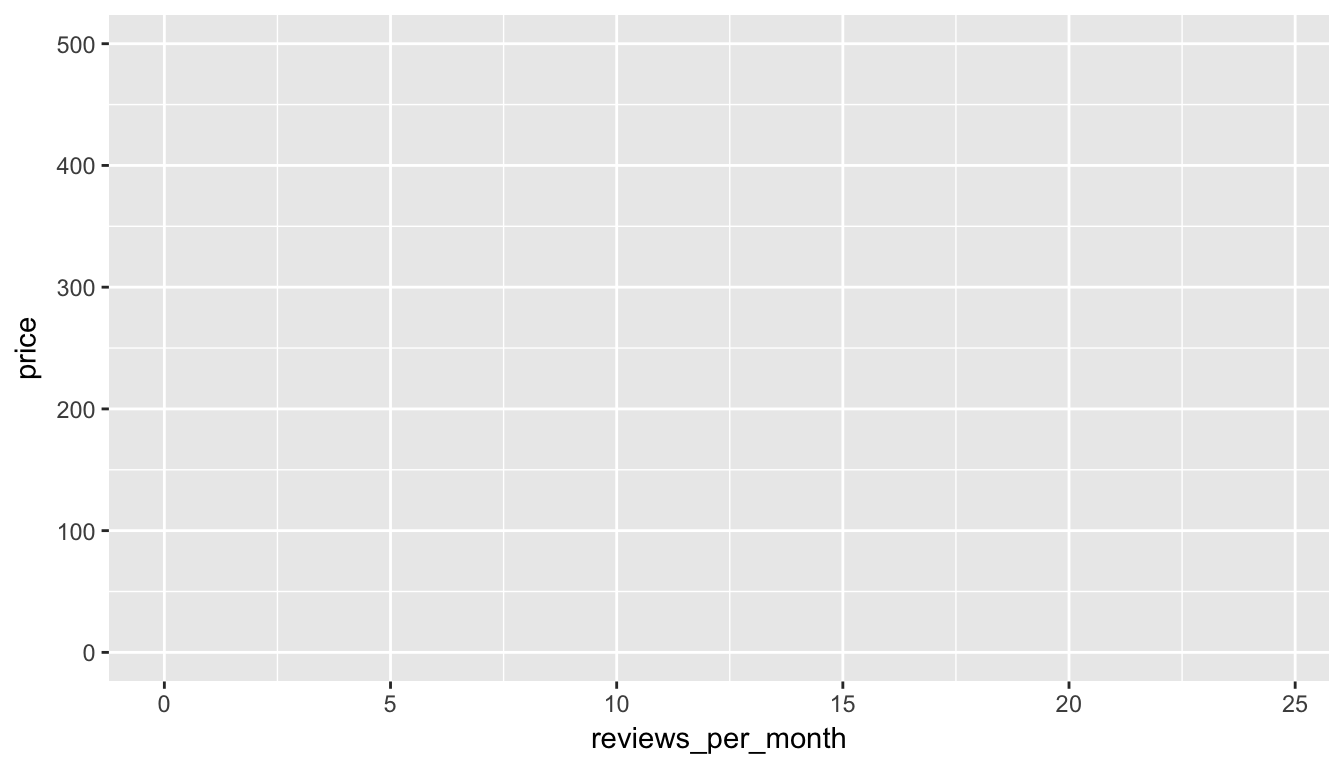• Display the data or the statistical summaries of the data

• Mainly use geom_xxx() function

• An alternative way is stat_xxx() function

• A plot must have at least one geom or stat function. there is no upper limit. You can add a layer to a plot using the + operator

• All kinds of geom function and stat function: ggplot2 cheat sheet

## Geom_xxx()

```ggplot(Airbnb, aes(price, reviews_per_month)) +
geom_point(size = 0.1) +
facet_grid(~room_type)```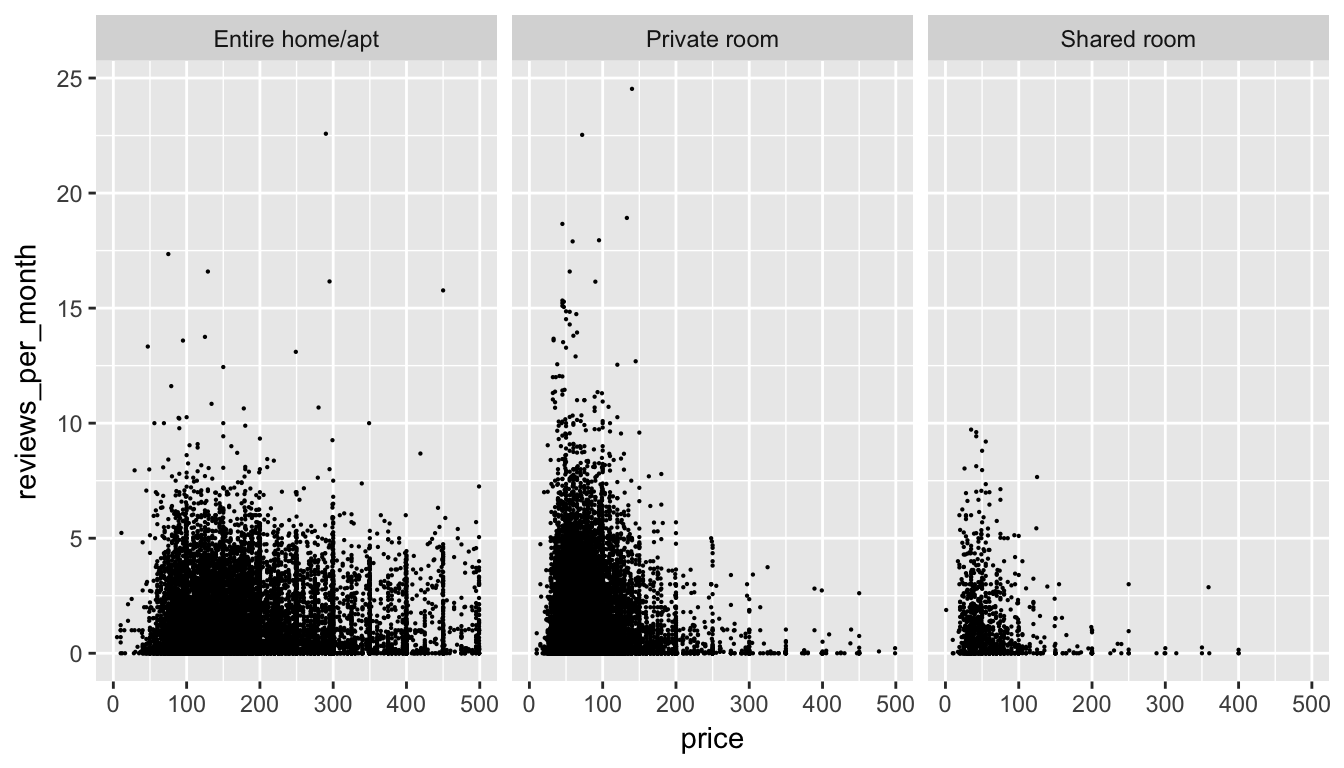## Aesthetic Mapping

• Describe how variables are mapped to visual properties

• aes()

• Specifying the Aesthetics in the Plot(ggplot()) or in the Layers(geom_xxx() or stat_xxx())

• Aesthetic Mapping can consists of Position (i.e., on the x and y axes), color (“outside” color), fill (“inside” color), shape (of points), linetype and size, etc.

## Aesthetic Mapping

```ggplot(Airbnb, aes(x = price)) +
geom_histogram(bins = 40, aes(color = room_type), fill = "grey") ```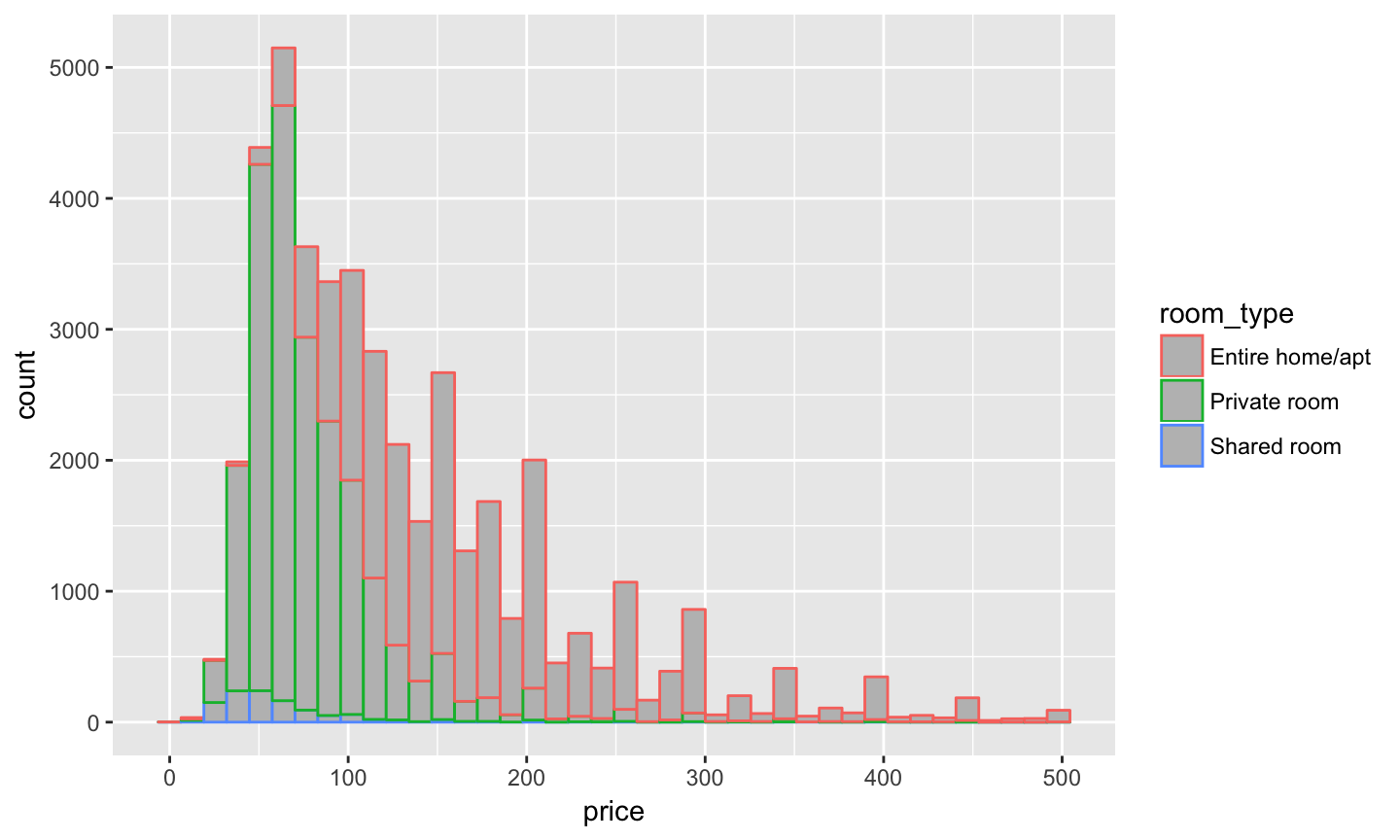## Aesthetic Mapping

```ggplot(Airbnb, aes(x = price)) +
geom_histogram(bins = 40, aes(fill = room_type), color = "grey") ```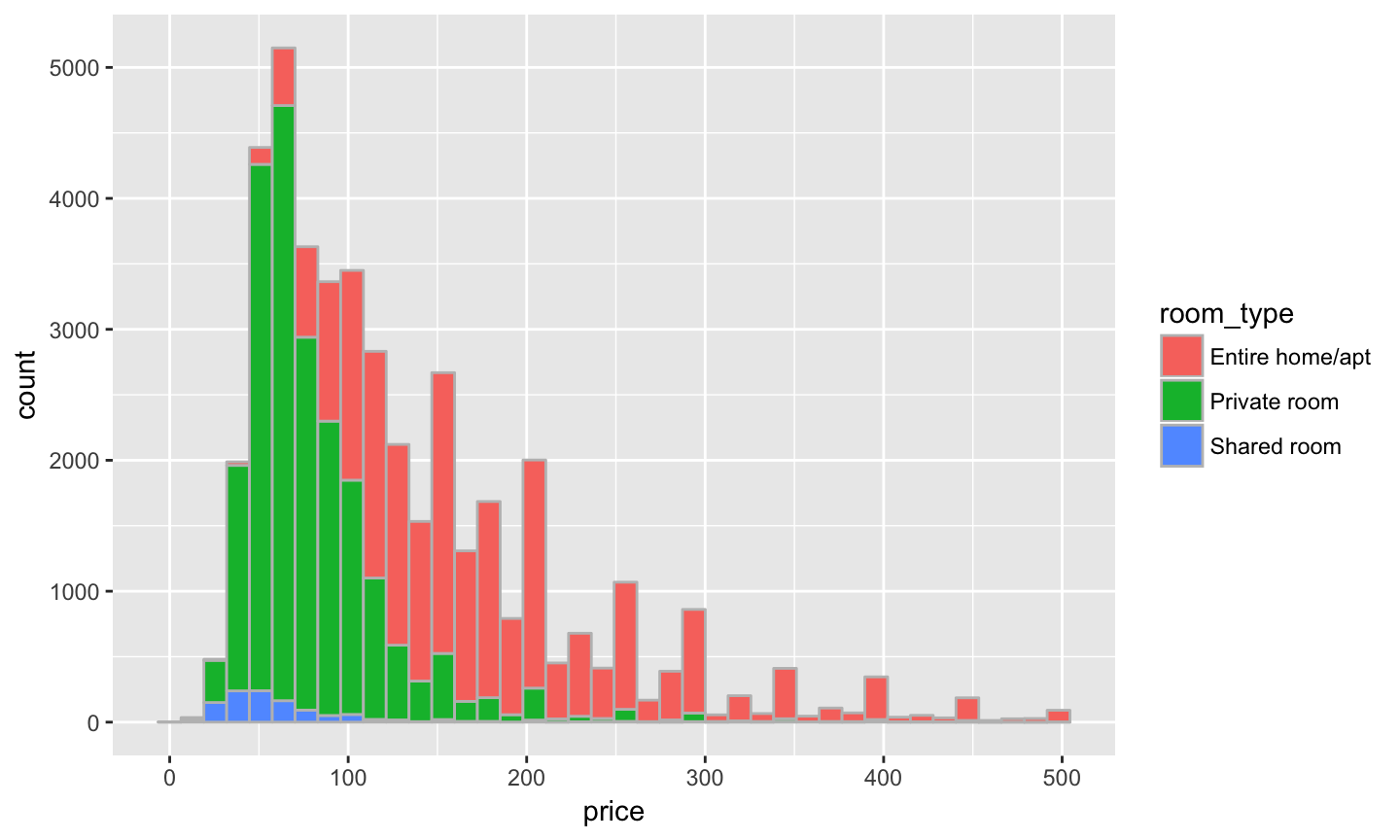## Construct Plot Layer by Layer

```ggplot(Airbnb) +
geom_violin(aes(neighbourhood_group, price), colour = 'blue')```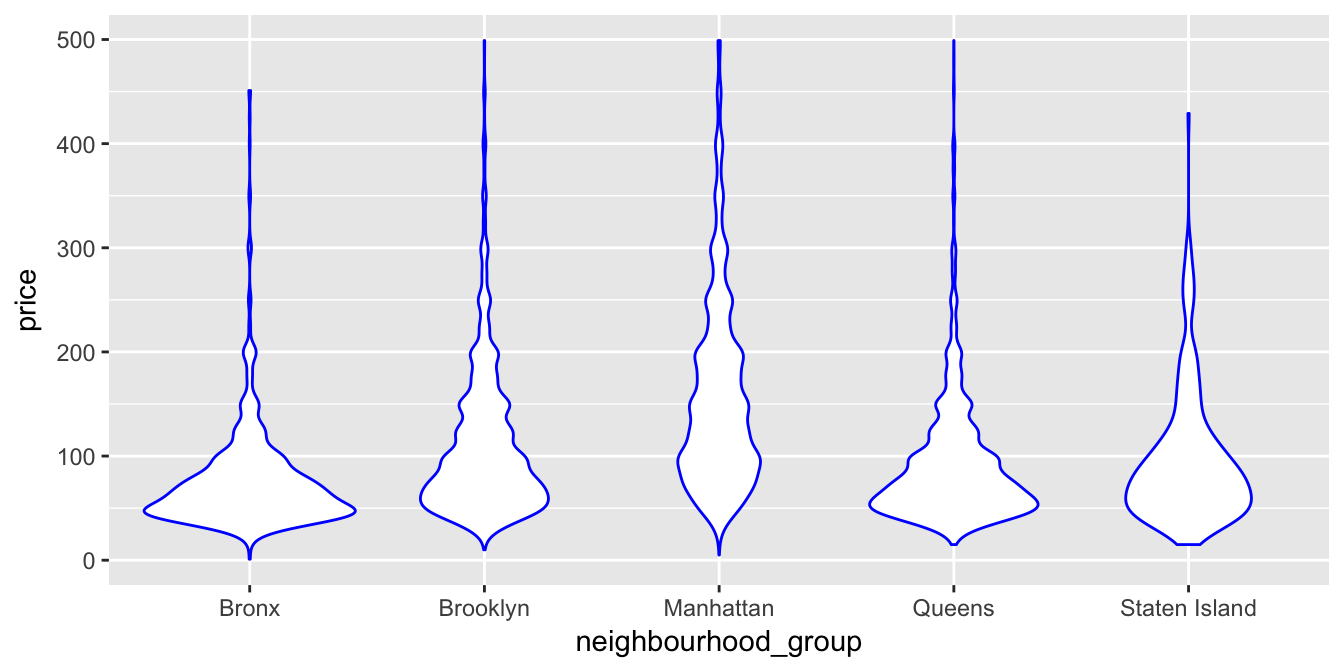## Construct Plot Layer by Layer

```ggplot(Airbnb) +
geom_violin(aes(neighbourhood_group, price), colour = 'blue') +
geom_boxplot(aes(neighbourhood_group, price), width = 0.16,
outlier.size = 0, notch = TRUE)```